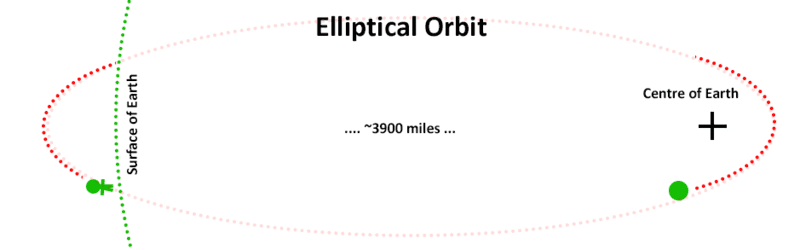# The Difference in Paths: Exploring Central Force & Gravity

• anuragchakraborty181
In summary, the ball follows an elliptical path due to the central force of gravity, while earth moves in an elliptical path around the sun due the same force of gravity. However, these paths are different due to the different approximations of gravity being a central force.f

#### anuragchakraborty181

Misplaced Homework Thread -- Moved to the School Forums
When we throw a ball in a projectile motion, the ball follows a parabolic path due to gravity. And we see that earth moves in an elliptical path around the sun due the same force of gravity. So why two paths are different due to the same force?
Explain using the idea of central force

•String theory guy
Quite simple. When we throw a ball in a projectile motion (and assuming no friction,), the ball follows an elliptical path, just like the satellite, and not a parabolic path like you'd get from an accelerating flat surface instead of gravity from a planet.

•topsquark
When we throw a ball in a projectile motion, the ball follows a parabolic path due to gravity. And we see that earth moves in an elliptical path around the sun due the same force of gravity. So why two paths are different due to the same force?
Explain using the idea of central force
Is this a homework question for you?

•topsquark
Very interesting question!
My guess is that both follow an elliptical path. The parabolic path being just a simplification for a flat earth.
A similar simplification is done for potential energy: pe = mgh
If one leaves the earth, the equation is no longer valid, as gravity is not a constant.
It's interesting how many of these there are. e = mc^2 is also a simplification of a more complicated equation.

•Orodruin
The earth is large compared to how far you can throw a ball. So we can assume that the direction of the gravitational force is constant, like a flat earth model with gravity always normal to the earth's flat surface. Then the math works out to a parabolic path.

But for objects that are far away from the earth, like the moon, the direction of the force of gravity from the earth changes throughout the moon's path, always pointing towards the center. In that case the math works out to elliptical paths.

Another way of thinking about this is that a parabola can be a good approximation of part of an elliptical curve for a small enough portion of that curve.

•topsquark, Rive and String theory guy
When we throw a ball in a projectile motion, the ball follows a parabolic path due to gravity.
Well, actually it's on an elliptical path around Earth: it's just Earth is not ideal enough for that and gets in the way for most of the path.

•DaveC426913 and topsquark
The more vertical you throw the ball the more parabolic the path is ; the more horizontal you throw the ball the more elliptical the path becomes. This gets messed up a bit by the Earth's rotation, of course, which induces a Coriolis effect.#### Attachments

•Orodruin
The more vertical you throw the ball the more parabolic the path is ; the more horizontal you throw the ball the more elliptical the path becomes. This gets messed up a bit by the Earth's rotation, of course, which induces a Coriolis effect.
The path is still elliptical in a non-rotating frame.

Of course, as has been said many times over, the path is parabolic only in the approximation that the gravitational field is constant. Furthermore, the path is elliptical only in the approximation of having a Kepler potential, ie, outside a spherically symmetric mass distribution (which the Earth and Sun are to quite good approximation—but not exactly and therefore the orbits are not exact ellipses)

... and then there is the gravitational force of all the other bodies in the solar system.

Not to mention for a projectile ignoring air resistance.

•jbriggs444
... and then there is the gravitational force of all the other bodies in the solar system.

Not to mention for a projectile ignoring air resistance.
Indeed. Compare the error from assuming constant gravity to the error from assuming zero air resistance. If one were going to refine the parabolic approximation, it would be efficient to worry about the largest errors first.

Alternately, if one had a strong enough arm to hurl a baseball at escape velocity, a parabolic trajectory would actually result, were it not for that pesky atmosphere.

•PeroK
Alternately, if one had a strong enough arm to hurl a baseball at escape velocity, a parabolic trajectory would actually result, were it not for that pesky atmosphere.
Parabolic? Hyperbolic?

Alternately, if one had a strong enough arm to hurl a baseball at escape velocity, a parabolic trajectory would actually result, were it not for that pesky atmosphere.
Assuming the exact escape velocity. Any faster and it's a hyperbola.

•jbriggs444 and DaveC426913
Post #1 tells us to explain using "the idea of central force". I don't see that an idea can explain anything.
We can note that the parabolic approximation overlooks that gravity is a central force, so is likely to produce a different answer. Don’t see that any more can be said in that respect.